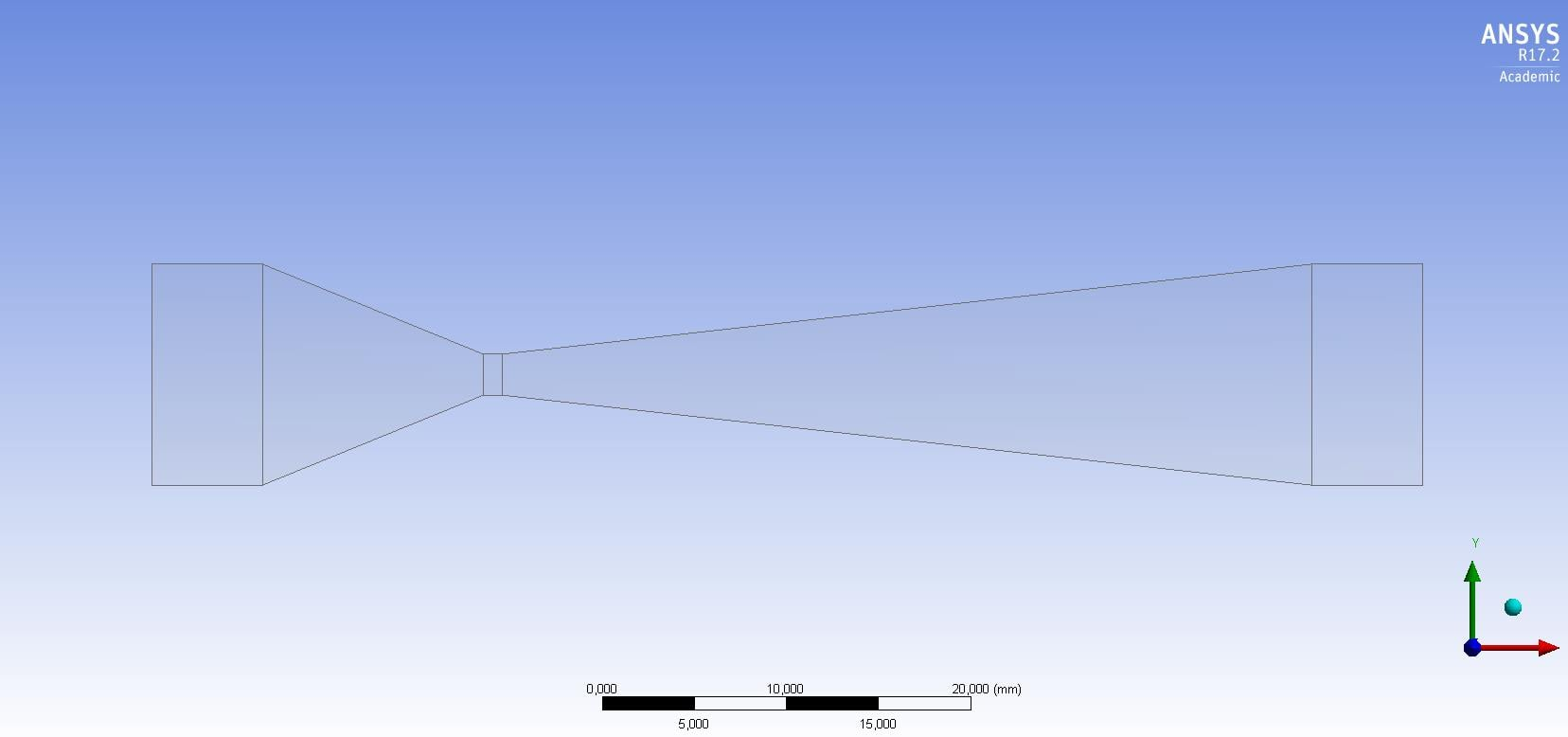# How to calculate the Y+ for a Venturi

Hello Everyone!

So, I am doing some simulations on hydrodynamic cavitation in a Venturi type tube and would like to find a good mesh for this, within the constraints of the student package. I would like to have a notion of how to find the best values for cell size near the wall.

What would be best? Do an analytical calculation in the Venturi regions and apply for each zone or calculate for the most critical zone and define for all? (since if the boundary layer is resolved in the critical zone, the same value would also satisfy the Venturi input for example and other regions). In this case, I say analytical calculation, to get a sense of the velocity in the regions and apply it to the Y + formula with the flow conditions, using Y + calculators available on CFD sites.

Below a image of geometry.Thanks a lot! Every help and idea are welcome.

Mantovani.

• edited May 2018

It largely depends on the turbulence model you are planning to use. I'd use the SST model focusing on the diverging section of the venturi. Ideally, you'd want the y+ to be less than 1 so that you can accurately predict the separation. But as you mention with the student package, you might have some trouble meeting that requirement depending on the flow velocity. I'll add more information to this post in a couple of days.

• edited May 2018

As Rahul mentioned, it depends on 2 factors:

1. Are you interested in the friction losses? (I would assume you are)

2. What Model are you using?

I found this mini-article which could help:

https://www.computationalfluiddynamics.com.au/turbulence-part-3-selection-of-wall-functions-and-y-to-best-capture-the-turbulent-boundary-layer/

Generally speaking:

-using models with enhanced wall treatment, Y+ value should be on the order of 1

- using wall function models Y+ value should be >15 to 300

I recommend you to read the article I attached.

• edited May 2018

It's very well guys.

As Rahul and Raef said, I saw in a article about the effect of cavitation hydrodynamic simulation using ANSYS, the author has tested 3 models of turbulence (k-epsilon, SST and Reynolds Stress) and the best result was the one that converged with the experimental situation (of course, using the same parameters and geometry) is the k-epsilon model.

I stay interested in capture the bubbles collapse, that is, I want be a best way to capture the cavitation hydrodynamic phenomenon within the constraints of the student package.

I decided to ask this question here because the flow through this type of geometry has in each region a peculiar behavior, even more in the divergent region where such an effect occurs.

So, Now I'll read the article gived by Raef.

Thanks one more time!